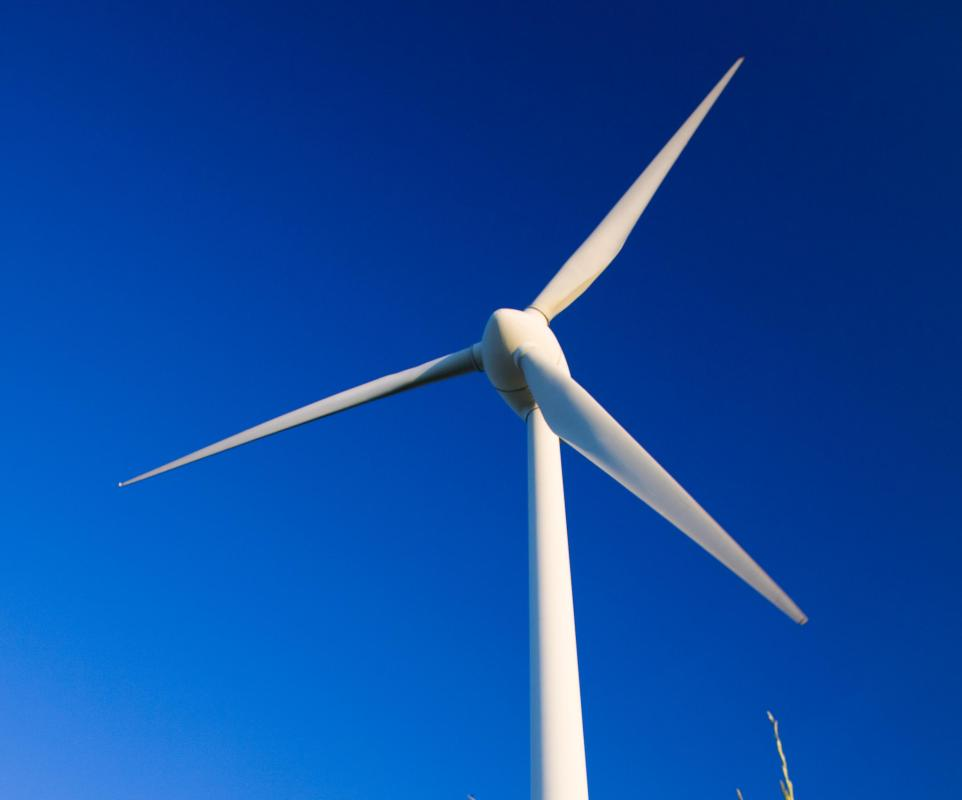# What Is Energy Conversion Efficiency?

Daniel Liden
Daniel LidenWind turbines convert wind to electricity. The can be less efficient when the wind is calm.

"Energy conversion efficiency" is a term used to describe the amount of useful energy output that results from a given energy input in a device used to convert energy from one form to another. A solar energy converter, for instance, converts radiant energy from the sun into usable electricity that can be used to power electrical devices. The ratio of the energy output to the energy input of this process describes the overall energy conversion efficiency of the solar energy converter. Knowing the efficiency is important if one wants to estimate the amount of useful output received from a given conversion process or device. In some cases, the overall efficiency over time will be affected by factors unrelated to the device; a solar energy converter, for instance, only works during the day and is affected negatively by clouds.Fireplaces generally only heat the areas immediately around them, making them inefficient when compared with other types of heating systems.

A conversion device can lose efficiency through a variety of means. Some devices intended to convert one form of energy to another lose energy as heat. An engine intended to convert chemical energy to work or motor power may, for instance, release some energy as heat. This heat does not contribute to the desired output of work, so it is waste energy. As such, it detracts from the overall energy conversion efficiency, because only the desired output is considered in the output-to-input ratio.

The energy conversion efficiency ratio of output to input is generally expressed as a percentage from zero to 100 percent or as a number from zero to one. This number does not have a unit or dimension; it is simply a ratio of the energy received from a conversion to the energy put into the conversion. A device or process with high energy-conversion efficiency will have a ratio closer to one or to 100 percent, while low energy-conversion efficiency is represented by a ratio closer to zero or to zero percent.

Energy conversion efficiency is generally only used to describe a device when it is running properly and in good circumstances. It must be remembered, however, that a variety of environmental factors can negatively impact overall efficiency, as can general degradation of equipment over time. Clouds can negatively impact the efficiency of a solar energy converter just as windless days can reduce the efficiency of energy windmills. Even a device with nearly perfect energy-conversion energy will be nearly useless if the source of energy input is simply unavailable.

## You might also Like